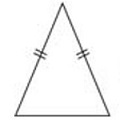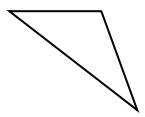# Classifying scalene, isosceles, and equilateral triangles by side lengths or angles

Triangles can be classified according to their sides or according to their angles. All of each side or angle may be of different or the same sizes; any two sides or angles may be of the same size.

The types of triangles classified by their sides are the following.

Equilateral triangle − A triangle with all three sides equal in length is an equilateral triangle. All angles of an equilateral triangle are equal too.

The slash marks indicate equal measure.Isosceles triangle − A triangle in which at least two sides are of equal length is an isosceles triangle.

An isosceles triangle also has two of its angles equal in measure.Scalene triangle − A triangle with all three sides of different lengths is a scalene triangle. The angles of a scalene triangle are also of different measure.Identify the given triangle as an equilateral, isosceles or a scalene triangle.### Solution

Step 1:

Given triangle has all sides of same length.

Step 2:

So given triangle is an equilateral triangle.

Identify the given triangle as an equilateral, isosceles or a scalene triangle.### Solution

Step 1:

Given triangle has sides of different lengths.

Step 2:

So given triangle is a scalene triangle.